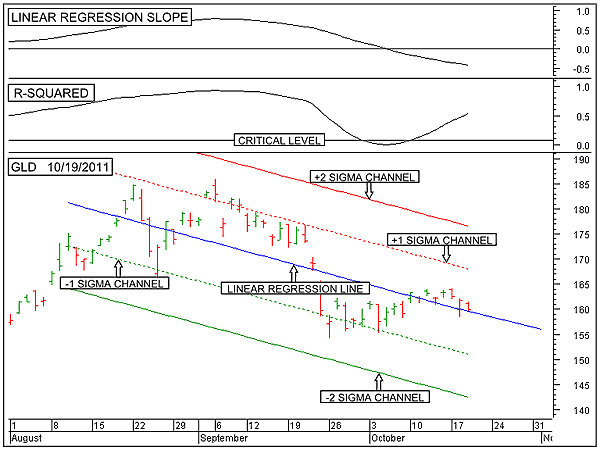HOT TOPICS LIST

INDICATORS LIST

LIST OF TOPICS

# Intermediate-Term Price Prediction For Gold

10/20/11 09:34:07 AM
by Alan R. Northam

This statistical analysis predicts the price of gold over the intermediate-term time period.

Security:   GLD
Position:   N/A

 Recently, I wrote an article that stated that gold remains in a long-term uptrend. In that article I also made reference that over the shorter term, gold was predicted to continue downward before the long-term upward rally would resume. I thought it fitting to show this shorter-term analysis. Statistical analysis can be used to measure differing parameters of the data collected. Within the analysis of the stock market, the data collected is price. By applying statistical analysis to price the trend, volatility, momentum, strength, and a prediction about the price movement can be determined. Here we will concentrate on making a prediction about the future price movement. The lower panel of Figure 1 shows the intermediate-term statistical analysis of the SPDR Gold Shares ETF (GLD). This figure shows the 50-day linear regression trendline (solid blue line), the upper one sigma channel line (dotted red line), the upper two sigma channel line (solid red line), the lower one sigma channel line (dotted green line), and the lower two sigma channel line (solid green line). Besides their statistical significance, the red channel lines act as resistance and the green channel lines support.FIGURE 1: GLD, DAILY. This chart shows the daily price chart of the SPDR Gold Shares ETF in the lower panel along with its upsloping 50-day linear regression trendline and its associated upper and lower channel lines. The top panel shows the linear regression slope indicator followed by the R-squared indicator. This chart shows that GLD remains in a intermediate-term downtrend. Graphic provided by: MetaStock. In making a prediction, the first thing that a statistician must calculate is the R-squared value. The R-squared value must be above its critical level before a reliable prediction about future price can be made. In the middle panel of Figure 1 is shown an indicator that measures the R-squared value. This indicator shows that it has moved above its critical level and is moving higher. The higher this indicator moves, the more reliable the prediction about price. With the R-squared value above its critical level, the statistician knows that a reliable prediction about the future price can be made. The statistician also knows that unless the R-squared value reads 0.0, price will not be exactly as predicted, but the higher the R-squared value, the closer price will be to the predicted value. More realistically, the price prediction is a guide as to the direction of price and is not an exact calculation.I should note that there are other methods of statistical analysis that can be used to determine the error in the future price prediction. This error can then be used to determine a predicted price range. With the R-squared value above its critical level, a valid linear regression trendline (solid blue line) can be drawn on the price chart and extended into the future, as shown in the bottom panel of Figure 1. This extended linear regression line is then used to predict price. As each trading session unfolds and new price data is added to the chart, the extended 50-day linear regression line is moved forward. As long as the R-squared value continues to remain above its critical value, the extended linear regression line will continue to predict future price. As long as the R-squared value continues to rise, price should continue to follow the predicted price. However, if the R-squared value starts to turn back down, the prediction of price will start to contain more and more error as the actual future price starts to move away from the linear regression line.As the R-squared indicator starts to turn down and price starts to move further away from its predicated value, the standard deviation channel lines come into play. A move beyond the one sigma channel lines signals increasing volatility. A move beyond the two sigma channel lines warn of a possible change in the trend, and a move beyond the three sigma channel lines signal that a change in trend is occurring. Predictions are just that -- predictions. They are not guarantees. Predictions are a guide for the trader to use in making future trading decisions. This statistical analysis shows that over the intermediate term, price is predicted to continue down. The first sign that this shorter-term downtrend is becoming exhausted will occur when price breaks out above the upper one sigma standard deviation channel line. A warning that a reversal back upward will come when price breaks out above the upper two sigma standard deviation channel line. Finally, a signal that a change in direction is taking place will occur when price breaks out above the upper three sigma standard deviation channel line.

Alan R. Northam

Alan Northam lives in the Dallas, Texas area and as an electronic engineer gave him an analytical mind from which he has developed a thorough knowledge of stock market technical analysis. His abilities to analyze the future direction of the stock market has allowed him to successfully trade of his own portfolio over the last 30 years. Mr. Northam is now retired and trading the stock market full time. You can reach him at inquiry@tradersclassroom.com or by visiting his website at http://www.tradersclassroom.com. You can also follow him on Twitter @TradersClassrm.

 Comments or Questions? Article Usefulness 5 (most useful) 4 3 2 1 (least useful)

Date:�11/01/11Rank:�5Comment: